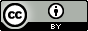### Management

```AddGeometryColumn
DropGeometryColumn
DropGeometryTable
postgis_full_version
postgis_geos_version
postgis_lib_version
postgis_proj_version
postgis_version
probe_geometry_columns
ST_SetSRID
UpdateGeometrySRID
```

--PostGIS tools --
```shp2pgsql
pgsql2shp

--PostgreSQL --
pg_dump
pg_restore
psql
```

### Meta Tables

```spatial_ref_sys
geometry_columns

```

### Geometry Creation

```ST_BdMPolyFromText
ST_BdPolyFromText
ST_GeomCollFromText
ST_GeomFromEWKB
ST_GeomFromEWKT
ST_GeomFromText
ST_GeomFromWKB
ST_MakeLine
ST_MakePolygon
ST_MakePoint
```

### Relationship

```ST_Contains*2
ST_CoveredBy1,2
ST_Covers1,2
ST_Crosses*2
ST_Disjoint*
ST_DWithin1,2
ST_Equals*
ST_Intersects*2
ST_Overlaps*2
ST_Relate*
ST_Touches*2
ST_Within*2```

### Spatial Aggregates

```ST_Accum
ST_Collect
ST_Extent
ST_Union*
ST_MakeLine
ST_MemCollect
ST_MemGeomUnion*
ST_Polygonize*
```

### Geometry Editors

```ST_AddBBOX
ST_Affine
ST_Collect
ST_DropBBOX
ST_Force_collection
ST_Force_2d
ST_Force_3d, ST_Force_3dm
ST_Force_3dz
ST_Force_4d
ST_LineMerge
ST_Multi
ST_RemovePoint
ST_Segmentize
ST_SetPoint
ST_SnapToGrid
```

### Linear Referencing

```ST_line_interpolate_point
ST_line_substring
ST_line_locate_point
ST_locate_along_measure
ST_locate_between_measures
```
PostGIS ver. 1.3.1 Quick Guide - Cheatsheet PDF VersionOfficial PostGIS Documentation URL: http://postgis.refractions.net/docs/
Refractions PostGIS Support and Training: http://www.refractions.net/products/postgis/
```This list is not comprehensive but tries to cover at least 80%.
We also leave out alternative names for functions.
The main difference between prior versions and 1.3.1 is that
in 1.3.1 and moving forward the MM naming convention of
prefixing using ST_ is in place.
The old names will still work, but moving forward you should use the ST_ names.
*Requires GEOS so in general slower than other functions
+Will use GEOS if compiled with GEOS
Most commonly used functions and operators Code snippet available
Measurement functions return in same units geometry SRID except
for the *sphere and *spheroid versions which return in meters```
`Denotes a new item in this version 1`
`Denotes now automatically uses spatial indexes 2`

## Geometry Types - WKT Representation

```POINT(0 0)
LINESTRING(0 0,1 1,1 2)
POLYGON((0 0,4 0,4 4,0 4,0 0),(1 1, 2 1, 2 2, 1 2,1 1))
MULTIPOINT(0 0,1 2)
MULTILINESTRING((0 0,1 1,1 2),(2 3,3 2,5 4))
MULTIPOLYGON(((0 0,4 0,4 4,0 4,0 0),(1 1,2 1,2 2,1 2,1 1)), ..)
GEOMETRYCOLLECTION(POINT(2 3),LINESTRING((2 3,3 4)))
```

## BBox Operators

These operators utilize indexes. They compare bounding boxes of 2 geometries
```A &< B (A overlaps or is to the left of B)
A &> B (A overlaps or is to the right of B)
A << B (A is strictly to the left of B)
A >> B (A is strictly to the right of B)
A &<| B (A overlaps B or is below B)
A |&> B (A overlaps or is above B)
A <<| B (A strictly below B)
A |>> B (A strictly above B)
A = B (A bbox same as B bbox)
A @ B (A completely contained by B)
A ~ B (A completely contains B)
A && B (A and B bboxes interact)
A ~= B  - true if A and B geometries are binary equal?
```

## Common Use SFSQL Examples

``` --Create a geometry column named the_geom in a
--table called testtable located in schema public
-- to hold point geometries of dimension 2 in WGS84 longlat
SELECT AddGeometryColumn('public', 'testtable', 'the_geom', 4326, 'POINT', 2);```
```--Insert a record into the new table
INSERT INTO testtable(description, the_geom)
VALUES('center of boston',
ST_GeomFromText('POINT(-71.0891380310059 42.3123226165771)', 4326));```
```--Insert a point record into the new table - faster than st_geomfromtext for points
INSERT INTO testtable(description, the_geom)
VALUES('center of boston',
ST_SetSRID(ST_MakePoint(-71.0891380310059, 42.3123226165771), 4326));```
```--Create a spatial index on the new geometry column
ALTER TABLE testtable ALTER COLUMN the_geom SET NOT NULL;
CREATE INDEX idx_testtable_the_geom ON testtable USING gist(the_geom);
ALTER TABLE testtable CLUSTER ON idx_testtable_the_geom;```
```--Find the neighborhood with the smallest area
SELECT neigh_name, ST_Area(the_geom)
FROM neighborhoods
ORDER BY ST_Area(the_geom) limit 1;```
```--Find the total area of each ward in square feet of wards in Boston,
--the extent (bounding box) of each ward, average sqft per precinct in each ward
SELECT ward, sum(ST_Area(ST_Transform(the_geom,2249))) as totarea,
avg(ST_Area(ST_Transform(the_geom,2249))) as avgarea_precinct,
ST_Extent(ST_Transform(the_geom,2249)) as wardextent
FROM wardprecincts WHERE city = 'Boston'
GROUP BY ward;```
```--Find all land parcels within 100 units of a specific parcel.
SELECT l2.parcel_id, l2.st_num, l2.st_name
FROM landparcels l , landparcels l2
WHERE ST_DWithin(l.the_geom, l2.the_geom, 100)
AND l.parcel_id = '1234560000';```
```--Break up multipolygons into individual polygons
SELECT neigh_name,
ST_GeometryN(the_geom, generate_series(1, numgeometries(the_geom))) As polygeom
FROM neighborhoods;
```
```--Take individual polygons and create one multipolygon for each neighborhood
--Note if you have a mixed collection of geometries, will return a geometry collection
SELECT neigh_name, ST_Collect(polygeom) as the_geom
FROM neighborhoods
GROUP BY neigh_name;
```

## Using Shape Dumper/Loader Commandline Tools

```Load data into PostgreSQL from ESRI shape file
shp2pgsql -s 4326 neighborhoods public.neighborhoods > neighborhoods.sql
psql -h myserver -d mydb -U myuser -f neighborhoods.sql

Exporting data from PostgreSQL to ESRI Shape file
pgsql2shp -f jpnei -h myserver -u apguser -P apgpassword mygisdb
"SELECT neigh_name, the_geom FROM neighborhoods WHERE neigh_name = 'Jamaica Plain'"

This work is licensed under a Creative Commons Attribution.

Feel free to use this material for private or commercial purposes, but we ask that you please retain the http://www.bostongis.com Boston GIS website link.
Boston GIS     Paragon Corporation
Postgres OnLine Journal PostGIS in Action```

### Accessors

```ST_Dimension
ST_Dump
ST_EndPoint
ST_Envelope
ST_ExteriorRing
ST_GeometryN
ST_GeometryType
ST_InteriorRingN
ST_IsClosed
ST_IsEmpty
ST_IsRing
ST_IsSimple
ST_IsValid
ST_mem_size
ST_M
ST_NumGeometries
ST_NumInteriorRings
ST_NumPoints
ST_npoints
ST_PointN
ST_SetSRID
ST_StartPoint
ST_Summary1
ST_X
ST_XMin,ST_XMax
ST_Y
YMin,YMax
ST_Z
ZMin,ZMax
```

### Measurement

```ST_Area
ST_Azimuth
ST_Distance
ST_distance_sphere
ST_distance_spheroid
ST_length_spheroid
ST_length
length3d_spheroid
ST_max_distance
ST_Perimeter
```

### Outputs

```ST_AsBinary
ST_AsText
ST_AsEWKB
ST_AsEWKT
ST_AsHEXEWKB
ST_AsGML
ST_AsSVG
```

### Geometry Processors

```ST_Boundary*
ST_Buffer*
ST_BuildArea*
ST_Centroid+
ST_ConvexHull*
ST_Difference*
ST_Expand
ST_ForceRHR
ST_Union*
ST_Intersection*
ST_PointOnSurface*
ST_Reverse
ST_RotateX
ST_RotateY
ST_RotateZ
ST_Scale
ST_Simplify
ST_SymDifference*
ST_Transform
ST_Translate
ST_TransScale
```